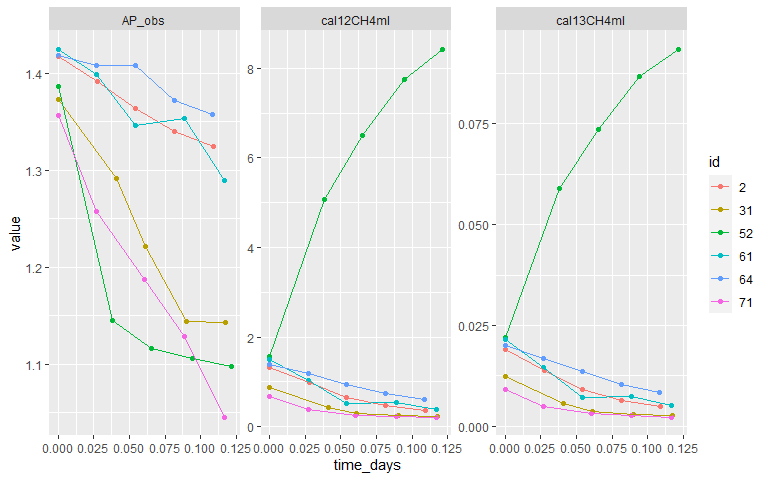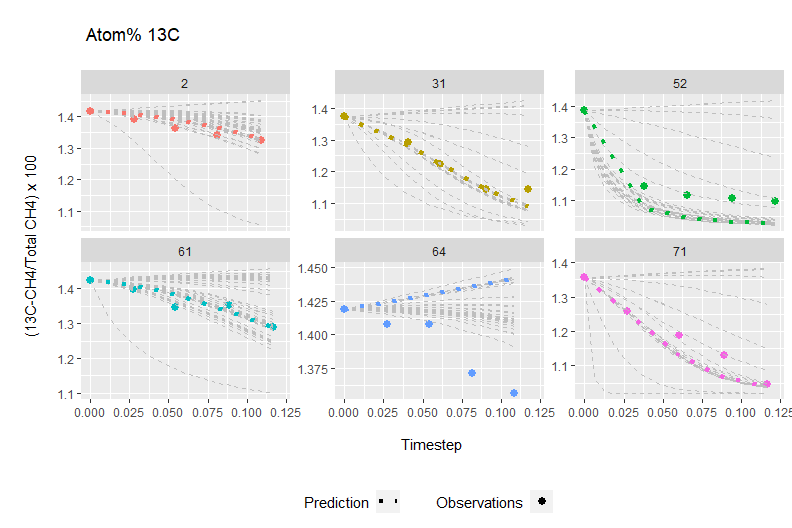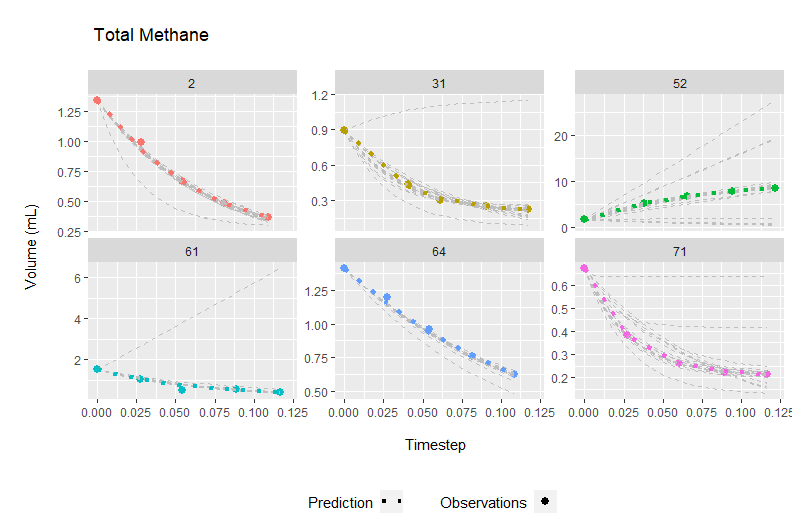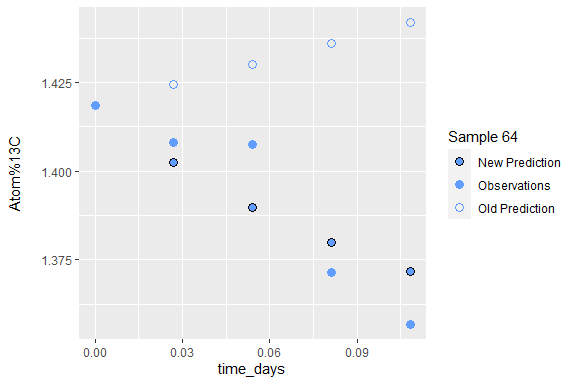# What is Pool Dilution?

Pool dilution is an isotope tracer technique wherein a biogeochemical pool is artificially enriched with its heavy isotopologue (molecules of the same chemical formula and bonding structure that differ only in their isotopic composition). After enrichment, the gross production and consumption rates of that pool can be calculated using the change in isotopic signature and absolute pool size over time. This technique can be applied to many different chemical species and was originally developed to measure soil nutrient transformations by Kirkham and Bartholomew in 1954.

## PoolDilutionR

PoolDilutionR takes time series data from isotopic enrichment experiments and optimizes the value of gross production, the first order rate constant of consumption, and/or fractionation to fit those data. The goodness of the fit is determined by the difference between observed and predicted values and weighted by both the quality of data (ie., instrunent precision) and the data explanatory power (ie., variation over time). This is accomplished using equations originally published in von Fischer and Hedin (2002), with some modifications. This approach differs from other pool dilution techniques in that it takes into account the effect of isotopic fractionation (see Under the hood. for more information.).

### Example Data

For this example we use one of the samples in the Morris2023 dataset that comes with PoolDilutionR. It consists of data collected at five time points during a methane pool dilution experiment (see ?Morris2023). These values have been converted from ppm to ml, although any single unit would suffice (ie., not a concentration).

Key to columns:

• id - sample identifier
• time_days - time (in days) between samples
• cal12CH4ml - mL’s of $$^{12}C$$-methane, calculated from incubation volume and sample ppm
• cal13CH4ml - mL’s of $$^{13}C$$-methane, calculated from incubation volume and sample ppm
• AP_obs - percent of methane that is $$^{13}C$$-methane, $$\frac{^{13}C}{(^{12}C+^{13}C)} * 100$$
library(PoolDilutionR)

OneSample_dat <- subset(Morris2023, subset = id == "71")
print(OneSample_dat)
#>    id  time_days cal12CH4ml cal13CH4ml   AP_obs
#> 26 71 0.00000000  0.6619600 0.00910000 1.356064
#> 27 71 0.02708333  0.3759093 0.00478686 1.257396
#> 28 71 0.06041667  0.2576457 0.00309738 1.187905
#> 29 71 0.08888889  0.2218850 0.00253422 1.129235
#> 30 71 0.11666667  0.2131561 0.00225264 1.045752

The question we are asking this data: What combination of gross fluxes (methane production and consumption) best fits the observed net fluxes implied by these concentration data?

### Solving for gross rates

To solve for gross methane production and consumption for this sample, we call pdr_optimize(), which in turn uses R’s optim() to minimize the error in the observed vs predicted pool size and isotopic composition. Errors are weighted by a combination of data quality and explanatory power (ie., cost_fuction()) and predictions are made using pdr_predict(). See Under the hood. for more information.

results <- pdr_optimize(
time = OneSample_dat$time_days, # time as a vector m = OneSample_dat$cal12CH4ml + OneSample_dat$cal13CH4ml, # total pool size n = OneSample_dat$cal13CH4ml, # pool size of heavy isotopologue
P = 0.1, # inital production rate for optim()
pool = "CH4", # indicates use of default fractionation constants for methane
m_prec = 0.001, # instrument precision for total pool size, as standard deviation
ap_prec = 0.01, # instrument precision for atom percent, as standard deviation
)
#> No frac_P provided; looking up from pdr_fractionation table
#> No frac_k provided; looking up from pdr_fractionation table
#> Estimated k0 = 11.873 from n_slope = -11.635

Notice that pdr_optimize() calculated or looked up three parameters that we didn’t provide: frac_P, the fractionation constant for production; frac_k, the fractionation constant for consumption; and k0, the initial value for the first order rate constant of consumption. The first two are taken from default values for methane as provided in the package’s pdr_fractionation dataset, while the latter is estimated from the data, using the slope of the pool size of heavy isotope over time (n_slope).

print(results)
#> $par #> P k #> 7.621946 36.495259 #> #>$value
#>  16.62139
#>
#> $counts #> function gradient #> 102 102 #> #>$convergence
#>  52
#>
#> $message #>  "ERROR: ABNORMAL_TERMINATION_IN_LNSRCH" #> #>$initial_par
#>        P        k   frac_P   frac_k
#>  0.10000 11.87255  0.01000  0.98000
#>
#> $initial_other #>$initial_other$method #>  "L-BFGS-B" #> #>$initial_other$lower #> P k #> 0e+00 1e-04 #> #>$initial_other$upper #> P k #> Inf Inf #> #>$initial_other$control #> list() pdr_optimize() returns a list with the following entries: • par - the final optimized values of (in this case) production (P) and the first order rate constant of consumption (k)*, as calculated by optim() • value, counts, convergence, message - these are all returned by optim() • initial_par - the initial parameters used for the model • initial_other - other settings passed to optim(); these typically include parameter bounds and optimization method *From k, the gross consumption rate can be calculated for any pool size of interest. This list has a great deal of detail, but we can also get a summarized form in a convenient data frame output format: pdr_optimize_df( time = OneSample_dat$time_days,
m = OneSample_dat$cal12CH4ml + OneSample_dat$cal13CH4ml,
n = OneSample_dat$cal13CH4ml, P = 0.1, pool = "CH4", m_prec = 0.001, ap_prec = 0.01 ) #> No frac_P provided; looking up from pdr_fractionation table #> No frac_k provided; looking up from pdr_fractionation table #> Estimated k0 = 11.873 from n_slope = -11.635 #> par value initial.P initial.k initial.frac_P initial.frac_k convergence #> 1 P 7.621946 0.1 11.87255 0.01 0.98 52 #> 1.1 k 36.495259 0.1 11.87255 0.01 0.98 52 ## Multi-sample Processing To demonstrate additional capabilities, we use the entire Morris2023 dataset: # create long data for plotting library(tidyr) long_Morris2023 <- pivot_longer(Morris2023, cols = cal12CH4ml:AP_obs) library(ggplot2) #> Warning: package 'ggplot2' was built under R version 4.2.2 ggplot( data = long_Morris2023, aes(time_days, value, color = id) ) + geom_point() + geom_line() + facet_wrap(~name, scales = "free_y")### Visual Summary of Multisample Output Now we show a graphical summary of the results from running these samples through pdr_optimize_df() with the same arguments as shown for a single sample above. samples <- split(Morris2023, Morris2023$id)

all_results <- lapply(samples, FUN = function(samples) {
pdr_optimize_df(
time = samples$time_days, m = samples$cal12CH4ml + samples$cal13CH4ml, n = samples$cal13CH4ml,
P = 0.1,
pool = "CH4",
m_prec = 0.001,
ap_prec = 0.01,
quiet = TRUE,
include_progress = FALSE
)
})
all_results <- do.call(rbind, all_results)

Below you can see the relative goodness of fit for final optimized P and k values (colored line) in terms of predicted isotopic signature (Atom% 13C) and pool size (Total Methane) for six samples. Grey lines represent values tested on the way to the final optimized fit. Code for reproducing these graphs is not shown, but is available in the publication associated with this package (citation and DOI pending acceptance).

#> Warning: Using size aesthetic for lines was deprecated in ggplot2 3.4.0.
#> ℹ Please use linewidth instead.Pool size predictions (below) are consistently tight to observations, whereas isotopic signature fits (above) have more variation.Poor fits can result from a variety of issues, including instrument error, low precision, and incorrect assumptions regarding fractionation. To help address the latter, pdr_optimize() allows users to choose up to four parameters (P, k, fractionation of P frac_p, fractionation of k frac_k) that pdr_optimize() will vary for optimizing model fit. Caution should be used in parameter selection, based on prior knowledge of the biological system.

## Variations with pdr_optimize()

### Optimization of Fractionation

Here we tell pdr_optimize() that the production and consumption values are known (and therefore fixed) and ask it to optimize only the fractionation rates:

# In this example, we assume that P and k are known, but that fractionation rates are unknown.

Sample64_dat <- subset(Morris2023, subset = id == "64")

new_fit <- pdr_optimize_df(
time = Sample64_dat$time_days, m = Sample64_dat$cal12CH4ml + Sample64_dat$cal13CH4ml, n = Sample64_dat$cal13CH4ml,
P = 8,
k = 1,
params_to_optimize = c("frac_P", "frac_k"),
m_prec = 0.001,
ap_prec = 0.01
)
#> No frac_P provided; looking up from pdr_fractionation table
#> No frac_k provided; looking up from pdr_fractionation table

newFitFracP <- new_fit[new_fit$par == "frac_P", ]$value
newFitFrack <- new_fit[new_fit$par == "frac_k", ]$value

# dataframe with new fit (optimizing P_frac and k_frac)
y_new <- pdr_predict(
time = Sample64_dat$time_days, m0 = Sample64_dat$cal12CH4ml + Sample64_dat$cal13CH4ml, n0 = Sample64_dat$cal13CH4ml,
P = 8,
k = 1,
frac_P = newFitFracP,
frac_k = newFitFrack
)

dat_new <- cbind(Sample64_dat, y_new)
dat_new$oldAPpred <- incdat_out[incdat_out$id == "64", ]\$AP_pred

# graph new fit
ggplot(data = dat_new) +
geom_point(aes(time_days, AP_pred, shape = "New Prediction", color = "New Prediction"),
size = 3, fill = "#619CFF"
) +
geom_point(aes(time_days, AP_obs, shape = "Observations", color = "Observations"),
size = 3
) +
geom_point(aes(time_days, oldAPpred, shape = "Old Prediction", color = "Old Prediction"),
size = 3
) +
scale_shape_manual(
name = "Sample 64",
breaks = c("New Prediction", "Observations", "Old Prediction"),
values = c("New Prediction" = 21, "Observations" = 16, "Old Prediction" = 1)
) +
scale_color_manual(
name = "Sample 64",
breaks = c("New Prediction", "Observations", "Old Prediction"),
values = c("New Prediction" = "black", "Observations" = "#619CFF", "Old Prediction" = "#619CFF")
) +
ylab("Atom%13C\n")print(new_fit)
#>        par      value initial.P initial.k initial.frac_P initial.frac_k
#> 1   frac_P 0.01292351         8         1           0.01           0.98
#> 1.1 frac_k 0.97710815         8         1           0.01           0.98
#>     convergence
#> 1             0
#> 1.1           0

(As before, we are using pdr_optimize_df() for tidy data frame output.)

We can see that

• the values of P and k were specified
• initial values for frac_P and frac_k were looked up from the package’s pdr_fractionation table based on the pool (here, methane)
• as requested, the optimizer only optimized frac_P and frac_k

The help page for pdr_optimize() provides other examples of this.

## Under the hood.

### Prediction

The equations in pdr_predict() come from the following equations in von Fischer and Hedin 2002.

Equation 5 describes the change in pool size over time in terms of $$P$$, gross production, and $$k$$, the first order rate constant of consumption.

$m_t = \frac{P}{k} - (\frac{P}{k} - m_0) * e^{(-kt)}$

Where:
$$m_t$$ is the total pool size at time t.
$$m_0$$ is the total pool size at time zero.

Equation 9 tracks the change in the heavy isotopologue over time in terms of consumption and its fractionation constant.

$n_t = n_0 * e^{(-k\alpha t)}$

Where:

$$n_t$$ is the pool size of the heavy isotopologue at time t.
$$n_0$$ is the pool size of the heavy isotopologue at time zero.

And:
$\alpha = \frac{k_{(\,^{13}C)\,}}{k_{(\,^{12}C)\,}}$
Where:
$$k_{(\,^{13}C)\,}$$ is the first-order rate constant for consumption of $$^{13}CH_4$$
$$k_{(\,^{12}C)\,}$$ is the first-order rate constant for consumption of $$^{12}CH_4$$.

In PoolDilutionR, we expand this equation to allow for the production of heavy molecules from authocthonous sources.

$n_t = \frac{p_{frac}}{k_{frac}} - ( \frac{p_{frac}}{k_{frac}} - 0) * e^{-k_{frac}t}$

Where $$k_{frac}$$ is equivalent to $$k*\alpha$$ above, for whatever heavy isotope is applicable.

The isotopic composition of the pool over time is described in Equation 10.

$AP_t = \frac {n_t}{m_t} + AP_p$

Which we can now simplify to:

Equation 10, adjusted $AP_t = (\frac{n_t}{m_t}) * 100$

Due to production of heavy isotopologue now being accounted for in our adjusted Equation 9.

Combined this looks like:

$AP_t = \left( \frac{\frac{p_{frac}}{k_{frac}} - ( \frac{p_{frac}}{k_{frac}} - 0) * e^{-k_{frac}t}} {\frac{P}{k} - (\frac{P}{k} - m_0) * e^{(-kt)}} \right) * 100$

### Cost Function

The pdr_cost() provides feedback to pdr_predict() on the quality of each iteration of fitted rates and/or fractionation constants. The sum of errors are weighted by the standard deviation of the observations, as well as a scaling factor, ($$N$$).

Equation 14:

$E = \left(\sum_{t=1}^j\frac {AP_{obs}(t) - AP_{pred}(t)}{SD_{obs-AP}}\right) * N_{ap} + \left(\sum_{t=1}^j\frac {m_{obs}(t) - m_{pred}(t)}{SD_{obs-m}}\right) * N_{m}$

Where:

$$SD_{obs-AP}$$ is the standard deviation among all observations of atom percent for a single sample
$$SD_{obs-m}$$ is the standard deviation among all observations of total pool size for a single sample

And:

$N_x = \frac{SD_{x_{observed}}}{SD_{x_{precision}}}$
Where:

$$x$$ is either atom percent ($$ap$$) or total pool size ($$m$$)
$$SD_{x_{precision}}$$ is the instrument precision for that variable as standard deviation (ie., standard precision)

Users have the ability to replace the default cost function (pdr_cost()) with their own, if desired.

### Fractionation

Fractionation describes the tendency of heavier isotopes and isotopologues to resist chemical transformation, whether these be phase changes or chemical reactions, whether spontaneous or enzyme-mediated. There are two major types of fractionation. Equilibrium fractionation occurs when phase changes favor the heavier isotopologue staying a lower energy state (Druhan, Winnick, and Thullner 2019, Urey 1947). A typical example would be the relative enrichment of the ocean in heavy water $$H_2^{18}O$$ due to preferential evaporation of light water $$H_2^{16}O$$. The second major type of fractionation is kinetic, and is classically associated enzyme selectivity. This is what drives the distinction in $$^{13}C$$ signatures between C3 and C4 plants (O’Leary 1981).

Because our knowledge of earth system processes and enzyme diversity is rapidly expanding, additional fractionation constants will be added to pdr_fraction as part of future package versions.

## Literature

• Druhan, J. L., Winnick, M. J., & Thullner, M. (2019). Stable Isotope Fractionation by Transport and Transformation. Reviews in Mineralogy and Geochemistry, 85(1), 239–264.
• Kirkham, D., & Bartholomew, W. V. (1954). Equations for following nutrient transformations in soil, utilizing tracer Data 1. Soil Science Society of America Journal. Soil Science Society of America, 18(1), 33.
• O’Leary, M. H. (1981). Carbon isotope fractionation in plants. Phytochemistry, 20(4), 553–567.
• Urey, H. C. (1947). The thermodynamic properties of isotopic substances. Journal of the Chemical Society, 0, 562–581.
• von Fischer, J. C., & Hedin, L. O. (2002). Separating methane production and consumption with a field-based isotope pool dilution technique. Global Biogeochemical Cycles, 16(3), 8-1-8–13.